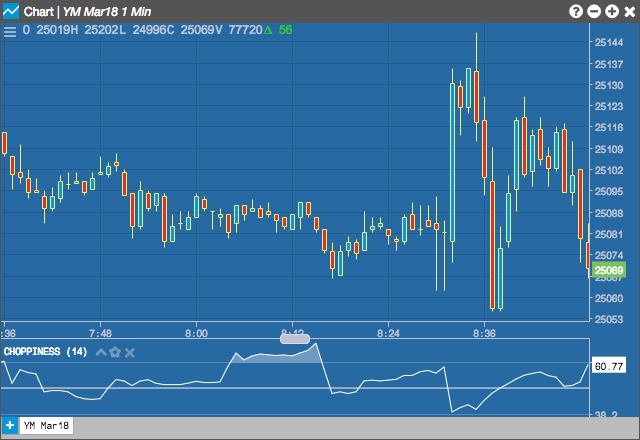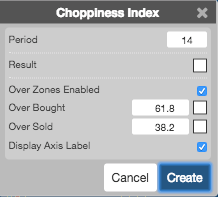Charts

# Choppiness Index

The Choppiness Index indicator helps determine whether the market is choppy (trading sideways) or not choppy (trading within a trend in either direction). It shows how long an individual segment of the market outperforms the market in general.## Configuration Options• Period: Number of bars to use in the calculations.
• Color Selectors: Colors to use for graph elements.
• Over Zones Enabled: Whether to shade the area between the plot and the horizontal overbought and oversold levels.
• Over Bought: Overbought quantity
• Over Sold: Oversold quantity
• Display Axis Label: Whether to display the most recent value on the Y axis.

## Formula

CI = 100 * LOG10( SUM(ATR(1), n) / ( MaxHi(n) - MinLo(n) ) ) / LOG10(n)

$CI = \frac{\frac{(100 * LOG10(\sum_1^n ATR)}{(MaxHigh(n) - MinLow(n))}}{LOG10(n)}$

where:

• n = User defined period length.
• LOG10(n) = base-10 LOG of n
• ATR(1) = Average True Range (Period of 1)
• SUM(ATR(1), n) = Sum of the Average True Range over past n bars
• MaxHigh(n) = The highest high over past n bars
• MinLow(n) = The lowest low over past n bars